Ummite Physics and Metaphysics:
"The Essential Texts"OUR THEORY OF UXGIIGIAM WAAM (SPACE).

When our brothers arrived in Earth year 1950 on OYAGAA (Earth), and after having learned the French language and gone for the first time to the library located at 58 rue Richelieu in Paris, they were surprised to read in the Earth mathematics texts in the library that, for instance, concepts like the POINT, the LINE and the PLANE continued to be considered by you as simple abstractions of an underlying reality of the UNIVERSE.

Thus when the mathematicians of the Earth define a point as a family of curves or as an ordered set of N numbers in an N space, they have the intuition of the structure of a scalar space with N dimensions in which the point is defined inside a frame of reference by its corresponding co-ordinates.

According to these ideas, a line will be a set of points with a one-to-one mapping with the set of real numbers, so that the distance between two points of a space Rn defined as basic, can remain defined.

Thus between two points A, B, of an N-space: D 59_ FIG 1being co-ordinates of two points A (X1, X2,X3... Xn), B (Y1, Y2, Y3... Yn), so that the framework of a multidimensional scalar space is thus defined.

This rigid model of the mathematics of Space does not satisfy a number of current physicists at all: that is true despite the fact that many others continue to accept the existence of this SPACE independent of the matter and the energy it contains. Then you invented another thing: the Space of the Phases. For you real space contains subatomic particles (another error as we will see further).

You postulate that each particle (NEUTRON, MESON, etc) must occupy in a given moment a position (POINT), but you must define the particle not only in terms of its position but also by its momentum.

Then you imagine an N-SPACE of six dimensions in which each particle is defined by six dimensions: D 59_ FIG 2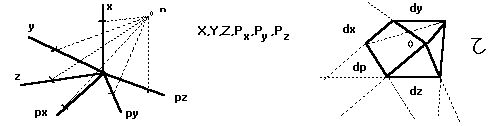You call this " entéléchie " space phases. You can then imagine an elementary volume made up of confined spaces, the limit of which would be a point. The elementary volume would be:

T = dx.dy.dz.dpx.dpy.dpz according to Heisenberg's uncertainty principle: dx.dpx > H; dy.dpy > H; dz.dpz > H, so that elementary volume will be T > H cubed.

(H is Planck's constant)

You name this elementary volume of order H cubed point of the space of the phases, since the infinitesimal point is shown when confronting intuition or physical significance, violating the uncertainty principle and since an elementary particle (electron, hyperon, neutrino, positron...) will be localised in an unspecified face of the elementary volume in question (phasic point) but never at the central point.

Our conception of UXGIGIAM (space) is radically different. Let us start with this concept of dimension which differs from the idea that you have made for yourselves. We will try to use symbols and concepts for your brothers who are unfamiliar the WUUA (mathematics)

You conceive of a UNIVERSE formed by a SCALAR SPACE i.e. : a space as it exists to our senses, in which the image of DIMENSION entails the image of a line or scalar. (D59_FIG3, F.1)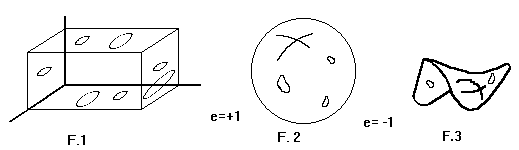Space, then, would be like an " IMMENSE VOLUME " and the COSMOS something like a SPHERE of positive (D59_FIG3, F2) or negative (D59_FIG3, F3) curvature.

The most intelligent among the Earth people suppose at least a curve inside the fourth dimension and identify the WAAM with RIEMANN's multidimensional space.

OUR UNIVERSE would then be like a positive or negative HYPERSPHERE but DIMENSION always being identified by you as a line or a scalar.

At the interior of this model of COSMOS you would put particles, atoms; forming Galaxies, gravity fields, magnetics and electrostatics, in short, Energy. (D59_FIG4).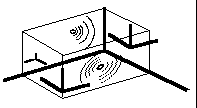D59_FIG4

We, on the contrary, know that the WAAM (cosmos) is composed of a network of IBIZOO UU. We conceive SPACE as an associated set of angular factors (D59_FIG5).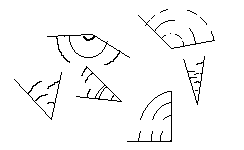D59_FIG5

For us the line in space does not exist, as we explain further, so the concept of OAWOO (dimension) takes on a different meaning for us. Such dimensions are associated not with scalar magnitudes (as the Earth people think), but on the contrary with angular magnitudes. (It is curious to note, for instance, that in their blindness, the physicists of the Earth do not give an angle a dimensional characteristic.)

If you were children in a school, perhaps we would use a rough example as a comparisons. The universe is like a "swarm of dragonflies " whose wings form different angles (D59_FIG6):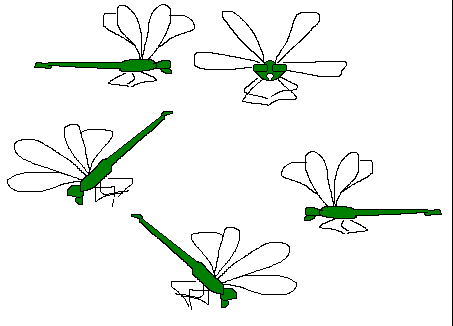D59_FIG6

All these dragonflies fly in such a way that not one has its wings oriented identically to any other. In other words, there will not be a single pair of dragonflies which, at a given moment, will be able to be superimposed so that the wings and the abdomens match exactly.. But, as we already said, this image is excessively simplified and distant in its analogy.

First of all each dragonfly occupies a place in space at each moment. I.e.: its inertia and centres of gravity occupy definite zones (according to this example).

An IBOZOO UU does not occupy any definite position, we cannot say of it that there is a probability of it being located at any one point.

In addition this flying insect has MASS and VOLUME, (at least in our minds) the IBOZOO UU is a particle without MASS or corporality. In a first conceptual approximation we could say of it that it is a set of oriented axes.

The angles formed by these axes are more important in such a set than the axes themselves (a mathematical fiction). (D59_FIG7)D59_FIG7

The dragonflies of our infinite swarm live in time, move in short intervals of time, infinitesimal distances. The IBOZOO UU does not exist in time, it IS time. (one of its angles is the magnitude TIME as we will explain it in another report with more explanations); to be more precise: what we call INFINITESIMAL INTERVAL OF TIME (dt) is only the difference in angular orientation between two dependent IBOZOO. (D59_FIG8)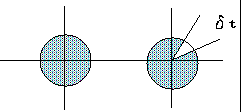D59_FIG8

If after this summary explanation you visualise our model of space by imagining for example that space is a " dense mass of particles similar to atoms " you are in error, since the particles of a gas such as you know it occupy probable positions in an enclosure, whereas that is not the case with the IBOZOO UU.

You should not either identify such a space with the ancient concept of ETHER thrown out by the theory of relativity, since the NETWORK OF IBOZOO UU is not at all an elastic medium in which are immersed the atoms of bodies.

You could also ask us: in relation to what universal reference axis are directed the angles of the IBOZOO UU? Naturally NOT WITH ANY. There is no axis of reference in the WAAM for that would suppose a real line in the COSMOS, and such a line, as we indicated, is a FICTION. We can only refer to the angle of one of the secondary axes of an IBOZOO UU compared to another, arbitrarily adopted as a reference. We inform you that you should not imagine that subatomic particles are immersed inside this set of IBOZOO UU. Quite simply because any particle (electron, meson or graviton) is necessarily an IBOZOO UU directed in a particular way compared to the others.

In conclusion : we also conceive of a space of N DIMENSIONS. MASS, for example, is also a " curve of this multidimensional space ". It is the same for DISTANCES within the WAAM. Only our concepts of magnitude, curvature and distance are radically different from those of the Earth people. Thus when we represent Space graphically as a Line, a Point, we do as you do because such images are familiar to us. But we know that they are pure fiction.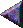Previous page

#### Synopsis